Electricity & Megnetism

# Magnetic field

A magnetic field is a region of space capable of exerting forces on moving electrical charges and on materials endowed withmagnetic properties . The magnetic field is a vector physical quantity measured in tesla (T) . Both the magnetic field produced by natural magnets and that generated by artificial magnets are the result of the movement of electrical charges inside the magnets.

## Definition of magnetic field

When an electrically charged particle moves, it creates a magnetic field. According to the laws of electromagnetism , this magnetic field originates from the variation of the electric field strength .

In magnetic materials, as in natural magnets, the magnetic field is the result of the alignment of a large number of magnetic domains, which are microscopic regions inside the magnet, endowed with a magnetic field, as if they were small compasses . The way the magnetic domains are organized defines what type of magnetism is present in the material.

In the following figure, we show how the magnetic domains of ferromagnetic, diamagnetic and paramagnetic materials are arranged, note:

Ferromagnetic materials are those that respond strongly to the presence of an external magnetic field; diamagnetic materials are repelled by the magnetic field; and finally, paramagnetic materials are slightly attracted by external magnetic fields.

Every magnetic field has two magnetic poles, called the south pole and the north pole. Both concern the direction of the magnetic field vector , which leaves and enters the north pole, as do the magnetic field definedlines , which will be later. Furthermore, it is not possible for a magnetic field to exist with only one of these poles, in respect of one of the laws of electromagnetism that predicts the non-existence of magnetic monopoles.

## magnetic field lines

In the figure we show the magnetic field lines, used as a device to facilitate the visualization of the field. Since the magnetic field is a vector quantity , each of the infinite points in space located in the vicinity of a magnet has a magnitude, a direction and a sense. Thus, representing it would be a very difficult task if it were not for the use of lines.

Magnetic field lines or induction lines are represented by the tangent of the magnetic field vector in that region of space. Let’s check their properties .

• They are always closed, since there is no magnetic monopole.
• They always emerge from the north magnetic pole and they always dip into the south magnetic pole, and the magnetic field vector always points towards magnetic north.
• Their density indicates the strength of the magnetic field in that region.
• They never cross.

## Magnetic field sources and formulas

There are different sources of magnetic field . They affect the way the field is distributed in space, so it is important to know some of them, as well as the formulas used to calculate them. We will start with the magnetic field produced by an electric current .

### Magnetic field generated by an electric current

When an electric current flows through a straight conducting wire, a circular magnetic field forms along its entire length. The induction lines of this field are concentric to the wire. Its direction is determined by the right hand rule, according to it, when we point the thumb in the direction of the electric current, the other fingers of the hand close in the direction of the magnetic field.

The magnetic field produced by an electric current, denoted by the symbol B , can be calculated by the following formula: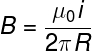B – magnetic field (T)

μ 0 – vacuum magnetic permeability (4π.10 –7 Tm/A)

i – electric current (A)

R – distance to wire (m)

### Magnetic field of a conducting loop

A loop is a closed conducting wire in a circular shape. The magnetic field produced in the central region of it can be calculated by the following formula , check: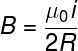### Magnetic field of a coil (solenoid)

Coils, also known as solenoids , are formed by a long conductor wire wound several times, being, therefore, the combination of a large number of turns.

The formula used to calculate the magnetic field inside the solenoid is this: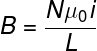N – number of turns of the solenoid

L – solenoid length

### Earth’s magnetic field

The Earth’s magnetic field originates from the relative motion of the Earth’s core and crust , as these structures rotate at different speeds. The presence of ions in the magnetic content of the Earth’s core and its rotation give rise to a three-dimensional magnetic field, which permeates the entire planet and protects our atmosphere , preventing it from being swept away by particles emitted by the Sun, known as the solar wind. The interaction between these particles and the Earth’s magnetic field gives rise to polar auroras .

Earth’s magnetic field lines emanate from a region near the geographic south pole , and so does the magnetic south pole, where the magnetic field lines merge. The magnetic and geographic poles do not coincide due to the Earth’s plane of rotation, which is different from the plane formed by the Equator .

## magnetic field exercises

Question 1) Calculate the intensity of the magnetic field produced by a straight conducting wire, carried by a constant electric current of 0.5 A, up to a distance of 50 cm from the wire.

Date : μ 0 = 4π.10 –7 Tm/A

a) 2.10 -7 T

b) 2π.10 –7 T

c) 5.0.10 -6 T

d) 2.5.10 -7 T

e) 4.0.10 -3 T

Template : letter b

Resolution:

Based on the data provided by the statement, we will calculate the magnetic field produced by the straight conductor, note: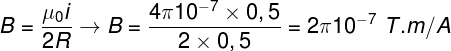After the calculation, we find that the magnetic field strength is 2π.10 -7 Tm/A.

Question 2) Based on your knowledge and according to the text, mark the correct alternative regarding the induction lines:

a) Induction lines are open lines, which leave magnetic north and enter magnetic south.

b) Induction lines leave the south magnetic pole and enter the north magnetic pole.

c) The induction lines are always closed and represent the tangent of the magnetic field vectors.

d) The concentration of induction lines in a region of space is inversely proportional to the intensity of the magnetic field in that region.

e) Magnetic field lines are used as a device to represent the magnitude, direction and direction of the magnetic field.

Template: letter c

Resolution:

We must remember that the lines of induction are actually the tangents of the magnetic field vectors. Furthermore, they are always closed, emerging from magnetic north and merging in magnetic south. Therefore, the correct alternative is letter c.

Question 3) An electric current of 1.0 A flows through a solenoid 10 cm long and 500 turns. Determine the strength of the magnetic field produced inside the solenoid.

Date : μ 0= 4π.10 –7 Tm/A

a) 2π.10 –3 T

b) 4π.10 –7 T

c) 4π.10 –3 T

d) 4.10 -4 T

e) π.10 –3 T

Template: letter a

Resolution:

Using the formula for the magetic field of the solenoid and based on the data provided in the statement, we must make the following calculation, note: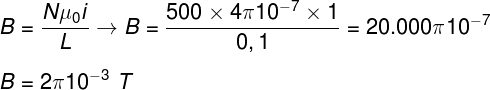The calculation shows that the intensity of the magnetic field produced inside the solenoid is equal to 2π.10 –3 T . Therefore, the correct alternative is letter a.